# 16. Interface and application programming¶

As always, there are many ways to approach this task. I decided to solve this task with processing and the Arduino ide, because I had no programming experience until the beginning of the fabacademy and this approach seems realistic to me for my time contingent.

Other options such as pyhon, java script or three. js require, from my point of view, greater prior knowledge.

I created the ldr as input for this assignment. When you change the brightness, the tree moves on the screen.
To achieve this, I have adapted some codes from Daniel Shiftman, Adrian Torres and from create.arduino.cc to my needs.

## the board¶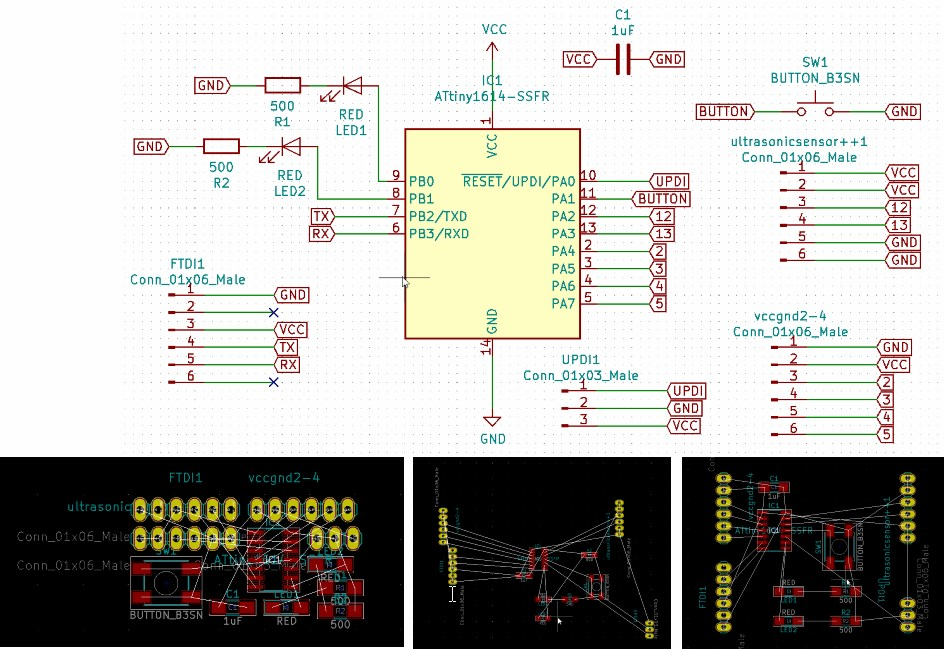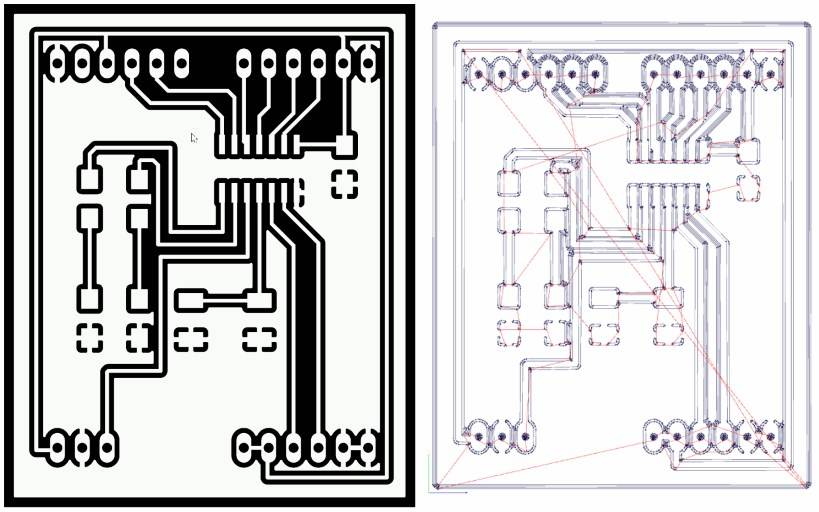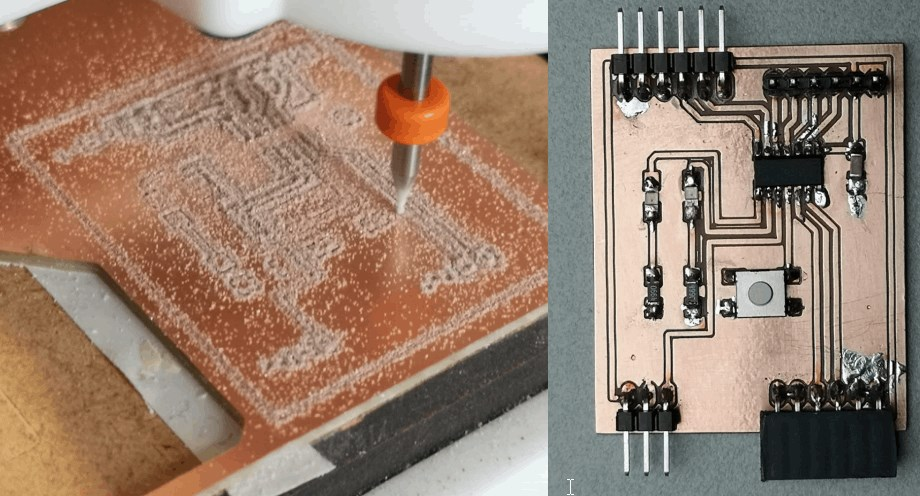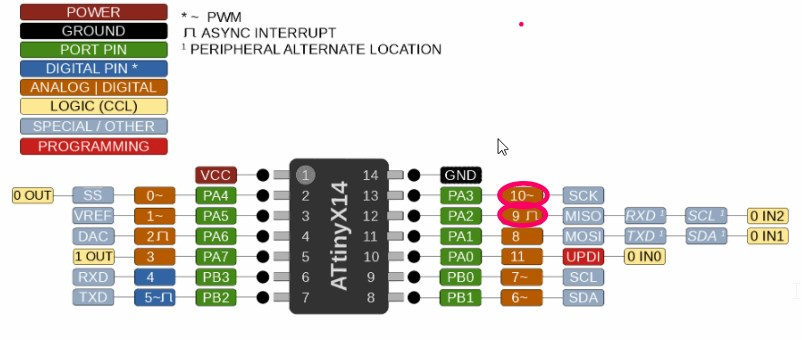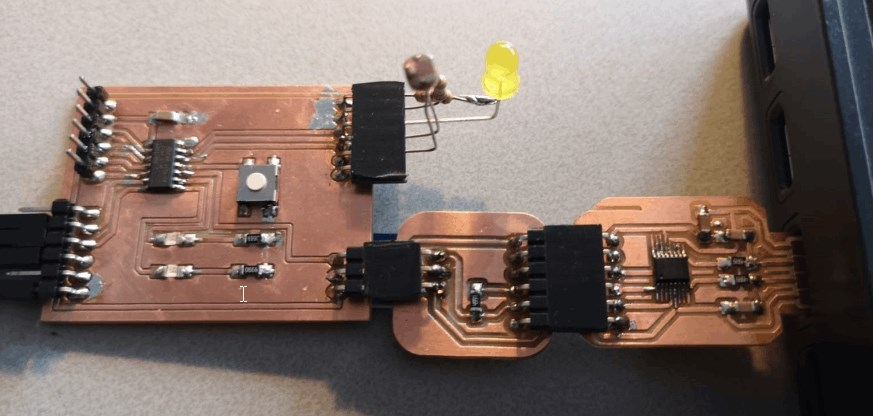After I adapted everything to my board, I uploaded the code via UPDI.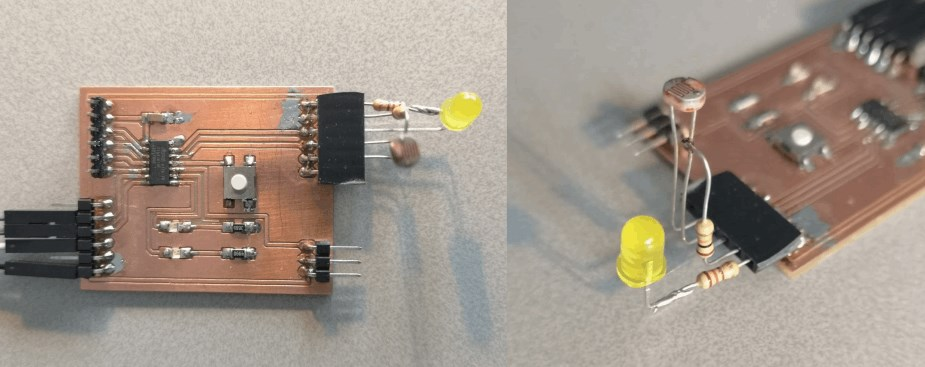and then the board is connected to the PC via FTDI in order to read out the data with the processing interface.

## code example¶

Processing

``````float theta;
import processing.serial.*;

float sensorValue; //variable for the serial data
Serial myPort;

void setup() {
size(640, 360);
myPort = new Serial(this, "COM5", 115200); // serial port
}

void draw() {
background(0);
frameRate(30);
stroke(255);
// Let's pick an angle 0 to 90 degrees based on the mouse position
float a = (sensorValue / (float) width) * 90f;
// Start the tree from the bottom of the screen
translate(width/2,height);
// Draw a line 120 pixels
line(0,0,0,-120);
// Move to the end of that line
translate(0,-120);
// Start the recursive branching!
branch(120);

}

void branch(float h) {
// Each branch will be 2/3rds the size of the previous one
h *= 0.66;

// All recursive functions must have an exit condition!!!!
// Here, ours is when the length of the branch is 2 pixels or less
if (h > 2) {
pushMatrix();    // Save the current state of transformation (i.e. where are we now)
rotate(theta);   // Rotate by theta
line(0, 0, 0, -h);  // Draw the branch
translate(0, -h); // Move to the end of the branch
branch(h);       // Ok, now call myself to draw two new branches!!
popMatrix();     // Whenever we get back here, we "pop" in order to restore the previous matrix state

// Repeat the same thing, only branch off to the "left" this time!
pushMatrix();
rotate(-theta);
line(0, 0, 0, -h);
translate(0, -h);
branch(h);
popMatrix();

println(sensorValue);
fill(0,0,0);// these are the colors inside
text(sensorValue + " " + "cm" , sensorValue, height/2);
textSize(32);
}
}void serialEvent(Serial myPort) { // sketch read the serial data
if (inString != null) {
inString = trim(inString);
float[] values = float(split(inString, ","));
if (values.length >=1) {
sensorValue = values; //first value in the list
}
}
}
``````

Board

``````const int ledPin = 10;
const int ldrPin = 9;

void setup() {
Serial.begin(115200);
pinMode(ledPin, OUTPUT);
pinMode(ldrPin, INPUT);
}
void loop() {
if (ldrStatus <= 500) {
digitalWrite(ledPin, HIGH);
Serial.println(ldrStatus);

} else {

digitalWrite(ledPin, LOW);
Serial.println(ldrStatus);
}
}
``````

## group¶

Last update: May 27, 2021